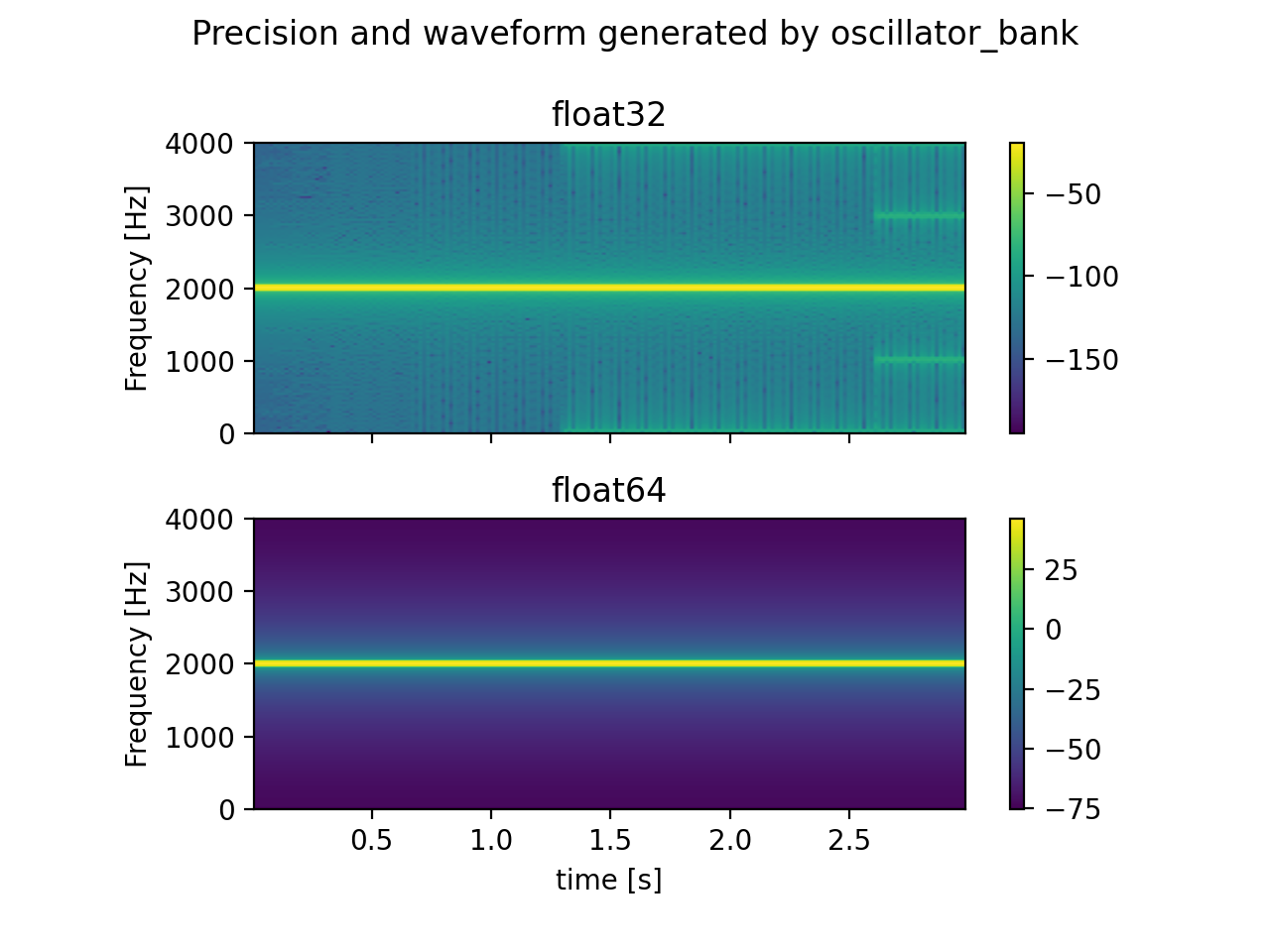Shortcuts

# torchaudio.prototype.functional.oscillator_bank¶

torchaudio.prototype.functional.oscillator_bank(frequencies: Tensor, amplitudes: Tensor, sample_rate: float, reduction: str = 'sum', dtype: = torch.float64) [source]

Synthesize waveform from the given instantaneous frequencies and amplitudes.Note

The phase information of the output waveform is found by taking the cumulative sum of the given instantaneous frequencies (frequencies). This incurs roundoff error when the data type does not have enough precision. Using torch.float64 can work around this.

The following figure shows the difference between torch.float32 and torch.float64 when generating a sin wave of constant frequency and amplitude with sample rate 8000 [Hz]. Notice that torch.float32 version shows artifacts that are not seen in torch.float64 version.Parameters:
• frequencies (Tensor) – Sample-wise oscillator frequencies (Hz). Shape (…, time, N).

• amplitudes (Tensor) – Sample-wise oscillator amplitude. Shape: (…, time, N).

• sample_rate (float) – Sample rate

• reduction (str) – Reduction to perform. Valid values are "sum", "mean" or "none". Default: "sum"

• dtype (torch.dpython:type or None, optional) – The data type on which cumulative sum operation is performed. Default: torch.float64. Pass None to disable the casting.

Returns:

The resulting waveform.

If reduction is "none", then the shape is (…, time, N), otherwise the shape is (…, time).

Return type:

Tensor

Tutorials using oscillator_bank:## Docs

Access comprehensive developer documentation for PyTorch

View Docs

## Tutorials

Get in-depth tutorials for beginners and advanced developers

View Tutorials